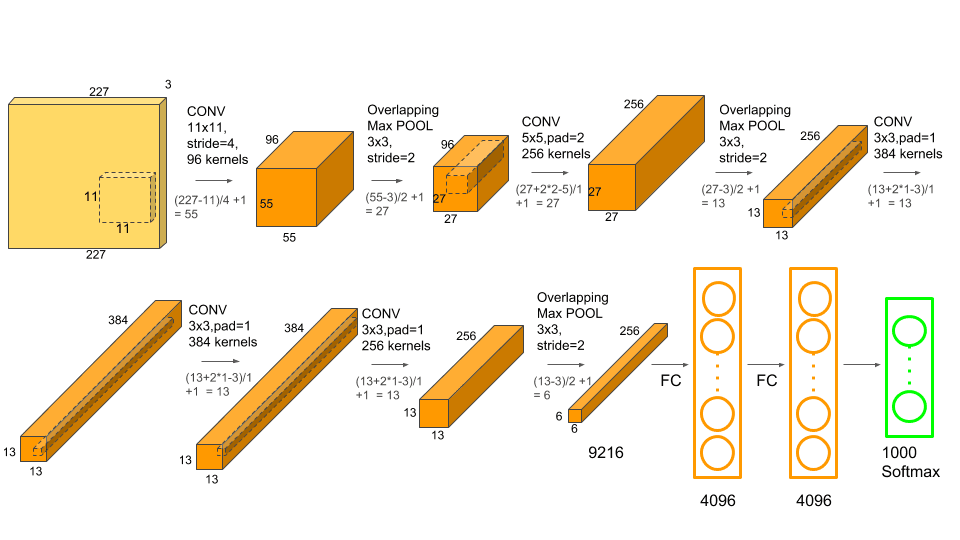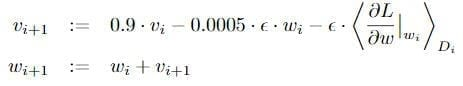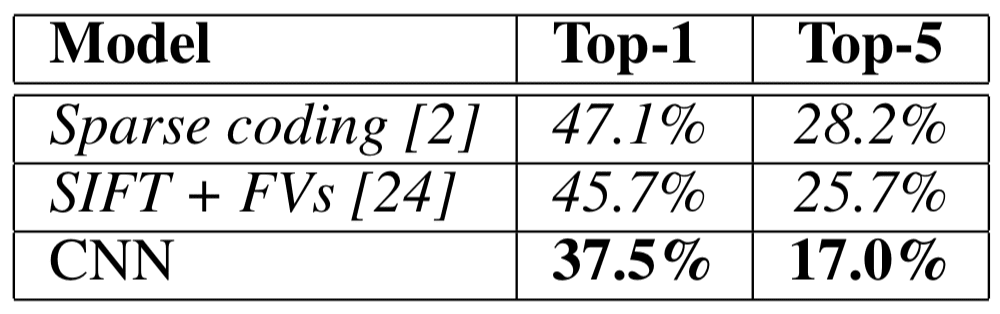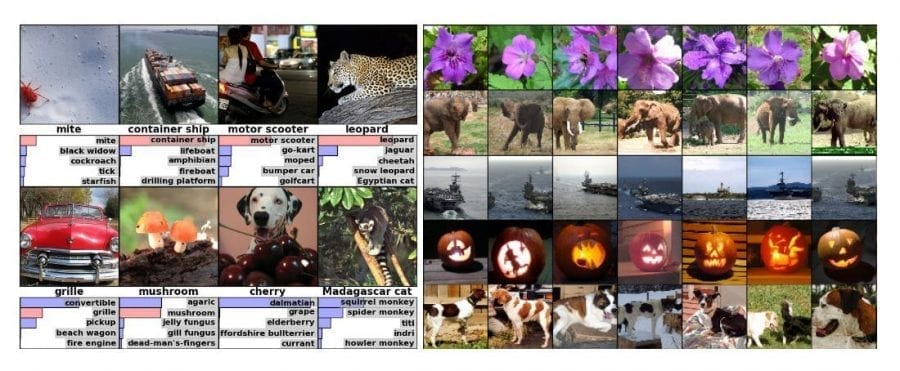• # AlexNet – ImageNet Classification with Deep Convolutional Neural NetworksAlexNet is the name of a convolutional neural network which has had a large impact on the field of machine learning, specifically in the application of deep learning to machine vision. It famously won the 2012 ImageNet LSVRC-2012 competition by a large margin (15.3% VS 26.2% (second place) error rates). The network had a very similar architecture as LeNet by Yann LeCun et al but was deeper, with more filters per layer, and with stacked convolutional layers. It consisted of 11×11, 5×5,3×3, convolutions, max pooling, dropout, data augmentation, ReLU activations, SGD with momentum. It attached ReLU activations after every convolutional and fully-connected layer. AlexNet was trained for 6 days simultaneously on two Nvidia Geforce GTX 580 GPUs which is the reason for why their network is split into two pipelines.

## Key Points

1. Relu activation function is used instead of Tanh to add non-linearity. It accelerates the speed by 6 times at the same accuracy.
2. Use dropout instead of regularisation to deal with overfitting. However, the training time is doubled with the dropout rate of 0.5.
3. Overlap pooling to reduce the size of the network. It reduces the top-1 and top-5 error rates by 0.4% and 0.3%, respectively.

## DataSet

ImageNet is a dataset of over 15 million labeled high-resolution images belonging to roughly 22,000 categories. The images were collected from the web and labeled by human labelers using Amazon’s Mechanical Turk crowd-sourcing tool. Starting in 2010, as part of the Pascal Visual Object Challenge, an annual competition called the ImageNet Large-Scale Visual Recognition Challenge (ILSVRC) has been held. ILSVRC uses a subset of ImageNet with roughly 1000 images in each of 1000 categories. In all, there are roughly 1.2 million training images, 50,000 validation images, and 150,000 testing images. ImageNet consists of variable-resolution images. Therefore, the images have been down-sampled to a fixed resolution of 256×256. Given a rectangular image, the image is rescaled and cropped out the central 256×256 patch from the resulting image.

## The Architecture

The architecture depicted in Figure 1, the AlexNet contains eight layers with weights; the first five are convolutional and the remaining three are fully connected. The output of the last fully-connected layer is fed to a 1000-way softmax which produces a distribution over the 1000 class labels. The network maximizes the multinomial logistic regression objective, which is equivalent to maximizing the average across training cases of the log-probability of the correct label under the prediction distribution. The kernels of the second, fourth, and fifth convolutional layers are connected only to those kernel maps in the previous layer which reside on the same GPU. The kernels of the third convolutional layer are connected to all kernel maps in the second layer. The neurons in the fully-connected layers are connected to all neurons in the previous layer.
In short, AlexNet contains 5 convolutional layers and 3 fully connected layers. Relu is applied after very convolutional and fully connected layer. Dropout is applied before the first and the second fully connected year. The network has 62.3 million parameters and needs 1.1 billion computation units in a forward pass. We can also see convolution layers, which accounts for 6% of all the parameters, consumes 95% of the computation.

### Training

AlexNet takes 90 epochs which were trained for 6 days simultaneously on two Nvidia Geforce GTX 580 GPUs which is the reason for why their network is split into two pipelines. SGD with learning rate 0.01, momentum 0.9 and weight decay 0.0005 is used. Learning rate is divided by 10 once the accuracy plateaus. The learning rate is decreased 3 times during the training process.The update rule for w was where i is the iteration index, is the momentum variable and epsilon is the learning rate. Equal learning rate for all layers, which was adjusted manually throughout training. The heuristic which was followed was to divide the learning rate by 10 when the validation error rate stopped improving with the current learning rate.

## Use-Cases and Implementation

The results show that a large, deep convolutional neural network is capable of achieving record-breaking results on a highly challenging dataset using purely supervised learning. Year after the publication of AlexNet was published, all the entries in ImageNet competition use the Convolutional Neural Network for the classification task. AlexNet was the pioneer in CNN and open the whole new research era. AlexNet implementation is very easy after the releasing of so many deep learning libraries.

## Result

The network achieves top-1 and top-5 test set error rates of 37.5% and 17.0%. The best performance achieved during the ILSVRC-2010 competition was 47.1% and 28.2% with an approach that averages the predictions produced from six sparse-coding models trained on different features, and since then the best-published results are 45.7% and 25.7% with an approach that averages the predictions of two classifiers trained on Fisher Vectors (FVs) computed from two types of densely-sampled features.
The results on ILSVRC-2010 are summarized in Table 1.(Left)Eight ILSVRC-2010 test images and the five labels considered most probable by the model. The correct label is written under each image, and the probability assigned to the correct label is also shown with a red bar (if it happens to be in the top 5). (Right) Five ILSVRC-2010 test images in the first column. The remaining columns show the six training images that produce feature vectors in the last hidden layer with the smallest Euclidean distance from the feature vector for the test image.
Subscribe
Notify of